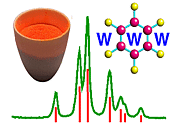Crystallite Size and Strain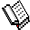Course Material Index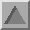Section Index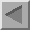Previous Page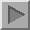Next Page

Crystallite Size and Strain

We now look in more detail at the sample broadening contribution. This arises from two main sources known as crystallite size and strain.

Crystallite Size

In 1918 Scherrer derived a formula relating the mean (volume average) crystallite size, L, of a powder to the broadening, β, of its powder diffraction peaks (ignoring other effects such as strain):

 BL = Kλ L cosθ

where θ is the usual Bragg angle, λ is the radiation wavelength, and K is a constant which depends on the assumptions made in the theory (e.g. the peak shape and crystallite habit, spherical crystallites being the easiest case to interpret) but is anyway close to unity and often taken as 0.9. Thus we see that β and L are reciprocally related: the greater the broadening the smaller the crystallite size and vice-versa. It is worth having a quick "intuitive glance" at why this should be so: In a previous section (Diffraction I) we showed how the interference from n = 2, 3, 4..... up to n = 1,000 and more, scattering centres produces fringes which ultimately become "diffraction" in three dimensions. These fringes start off being very broad at low n and become indefinitely sharp as n →∞. Another way of looking at this is to note that interference cannot occur if n = 1 and even the most primitive interference requires at least two centres (n = 2); then as n increases further the interference becomes more and more precise according to the number of contributing scatterers. In practice the sharpness eventually stops increasing when some other limit is reached, such as the inherent instrumental broadening, Binstrument, or a theoretical limit known as the Darwin limit. For real powder samples and diffractometers this limit is reached when the crystallite size, L, is around 1-10 μm (or larger). So the use of peak broadening to determine crystallite size is normally limited to cases where the average crystallite size is ≤ 1 μm.

Strain

The second main source of specimen broadening is strain, or more correctly inhomogeneous strain as we shall see. For the moment consider just one crystallite undergoing diffraction with the parameters d, λ, θ given by Bragg's Law, as illustrated in part (a) below: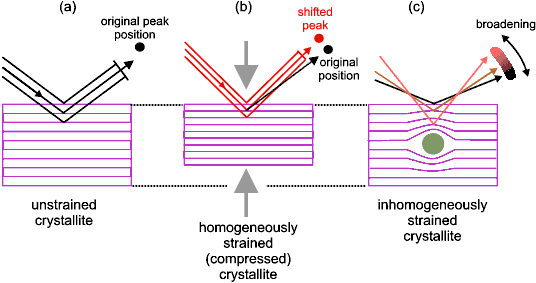If the crystallite is strained then the d spacings will be changed; a compressive stress would make the d spacings smaller (and a tensile stress would make the d spacings larger), say reducing a given spacing d to d− δd. Then by Bragg's Law the position of the peak will increase from 2θ to 2(+ δθ) where:

 λ = 2d sinθ λ = 2(d − δd) sin(θ + δθ)
If every crystallite in the sample were strained (compressed) by the same amount they would result in a peak shift from 2θ) to 2(θ + δθ as illustrated in part (b) of the above figure; i.e. there is only shifting of the diffraction peak, but no broadening. Indeed this shift can be exploited as an in-situ measurement of internal strain in objects such as railway tracks, as we shall see later in Modern Techniques & Applications II. We would describe such strain as homogeneous meaning that all crystallites were strained by the same amount. However if the strain is inhomogeneous then different crystallites will be strained by different amounts and the shifts in 2θ will be variable. For example a single diffraction spot (or line) would become a continuum of spots (or lines) the envelope of which in the limit of many crystallites would appear as just one smeared-out spot (or line); this is illustrated in part (c) of the figure for the case of a single crystallite containing a defect. Structural defects, such as interstitials, vacancies, dislocations and layer faults, induce inhomogeneous strain within a crystallite, the degree of strain obviously being greater at distances close to the actual defect. One can derive an approximate relationship relating the mean inhomogeneous strain (ε) to the peak broadening it produces (βε ). This is derived by differentiating Bragg's Law and relating the inhomogeneous strain to the differential δd/d . It has the simple form:

βε = Cε tanθ

where the value of the constant C depends on the assumptions made concerning the nature of the inhomogeneous strain, but is typically ≈ 4 or 5. You may have noted that the dependence of strain broadening on the Bragg angle, θ, is quite different from that with crystallite size. This difference is exploited as explained in the next part.Course Material IndexSection IndexPrevious PageNext Page
 © Copyright 1997-2006.  Birkbeck College, University of London. Author(s): Paul Barnes Simon Jacques Martin Vickers